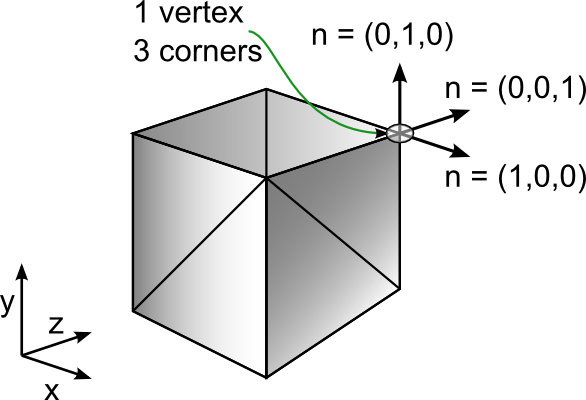# # Geometry data

The Simplygon API represents geometric objects using the GeometryData class. This class provides methods for accessing and manipulating geometric data.

## # Packed geometry data

Simplygon supports packed geometry data using the PackedGeometryData class. Packed geometry data stores geometry fields per vertex rather than per corner. The GeometryData class can return data in packed format using the NewPackedCopy method. Note that the packed geometry data will contain more vertices than original geometry due to vertices being split up because of non identical per vertex data. The PackedGeometryData class can return unpacked geometry data using the NewUnpackedCopy method. For more, see the packed geometry example.

## # Appending to geometry data

To combine two geometries, the AppendGeometry method of GeometryData, or the AppendPackedGeometry method of PackedGeometryData can be used. In the case of AppendPackedGeometry, it appends an GeometryData object to the packed geometry, using vertices from the packed geometry when available and adding new vertices from the appended geometry to the packed geometry if no matching vertices are found. It generates a vertex list describing the triangles of the appended geometry expressed in the vertex IDs from the combined vertex set of the packed geometry and the appended geometry.

A boolean flag determines if the method will collapse all vertices of the appended data to the closest matching vertex of the packed geometry, or allow new unique vertices to be added as required. Not allowing new vertices to be added could obviously produce strange meshes if the appended geometry is very different from the packed geometry it is appended to.

Used alongside the conservative setting of the DataCreationPreferences flag when using MeshLOD, AppendPackedGeometry produces a description of a generated LOD using the original vertices by appending the LOD to the packed original geometry. For a relevant example, check the data creation preferences example.

## # Geometry fields

Simplygon stores geometry information in a collection of arrays called fields. Several built-in fields are provided by the API. Users can also add their own custom fields to a geometry object. The geometry information in these fields are stored either on a per-vertex, per-corner or per-triangle basis.

A corner is a triangle-vertex pair so there are three corners for each of the triangles in the mesh. The default fields (apart from Coords) are mostly defined per-corner, to be able to define discontinuous data, such as normals and texture coordinates. The default geometry fields are listed below, they can all be used with Add[field name], Get[field name], Remove[field name];

Default field Tuple size Description
Vertex attribute
Coords 3 3D position values for each of the vertices in the geometry
BoneIds 1-4 Influencing bone identifier, see Working with skinning data
BoneWeights 1-4 Influencing bone weight, see Working with skinning data
VertexWeights 1 Importance value for feature preservation during reduction. See Working with vertex weights
VertexLock 1 Bool value to determine if vertex is locked. See Vertex-lock field
Triangle attribute
GroupIds 1 The group identifier for each of the triangles
MaterialIds 1 The material identifier for each of the triangles
Corner attribute
VertexIds 1 The vertex index used for that corner
Normals 3 Normal vectors for each of the corners in the mesh
TexCoords[level] 2 Texture coordinates for each corner in the mesh, per level. The level can be between 0 and SG_NUM_SUPPORTED_TEXTURE_CHANNELS - 1
Tangents[level] 3 Tangent vectors for each corner in the mesh, per level. The level can be between 0 and SG_NUM_SUPPORTED_TEXTURE_CHANNELS - 1
Bitangent[level] 3 Bitangent vectors for each corner in the mesh, per level. The level can be between 0 and SG_NUM_SUPPORTED_TEXTURE_CHANNELS - 1
Colors[level] 4 Color values for each corner in the mesh, per level. The level can be between 0 and SG_NUM_SUPPORTED_COLOR_CHANNELS - 1

## # Vertices and corners## # Vertex-lock field

The vertex lock field allows the user to fix certain vertices in the geometry so that they will not be modified by the simplification process. The vertex-lock field contains one Boolean value for each vertex that indicate whether the vertex should be locked or not.

### # Example - Adding a vertex-lock field

The following example shows how to add a vertex-lock field to a geometry object.

## # Working with vertex weights

The Simplygon API now provides the ability for the user to assign importance values per vertex as weights. These weights are used during reduction to keep some important features in a mesh intact (i.e facial features). This allows more artistic control during reduction process. A vertex weighting field has been added to the IGeometryData class. Use the AddVertexWeights method to add the field to an IGeometryData object.

The weight value should be a positive scalar between larger than zero, where small values means the vertices are less important to preserve. Values around 1.0 means they will be reduced just like normal. Large values will preserve the vertices accordingly.

### # Example - Adding vertex weights

This example shows how to add vertex weights.

## # User fields

The Simplygon API allows users to add their own fields to geometry objects. This information is preserved, if possible, when the geometry is processed by the various tools provided by the API.

There are three types of user fields, per-vertex, per-triangle and per-corner (triangle-vertex pair) fields. Vertex fields store a tuple for each vertex in the geometry, while per-triangle and per-corner fields store a tuple for each triangle and corner respectively. Vertex fields are added with the AddUserVertexField and AddBaseTypeUserVertexField, with their triangle and corner counterparts named AddUserTriangleField, AddBaseTypeUserTriangleField, AddUserCornerField and AddBaseTypeUserCornerField. This allows the user to either add pre-existing arrays or create new ones with a specific data type.

### # Example - Per-corner field

This example shows how to create and retrieve a new per-corner field containing real values.